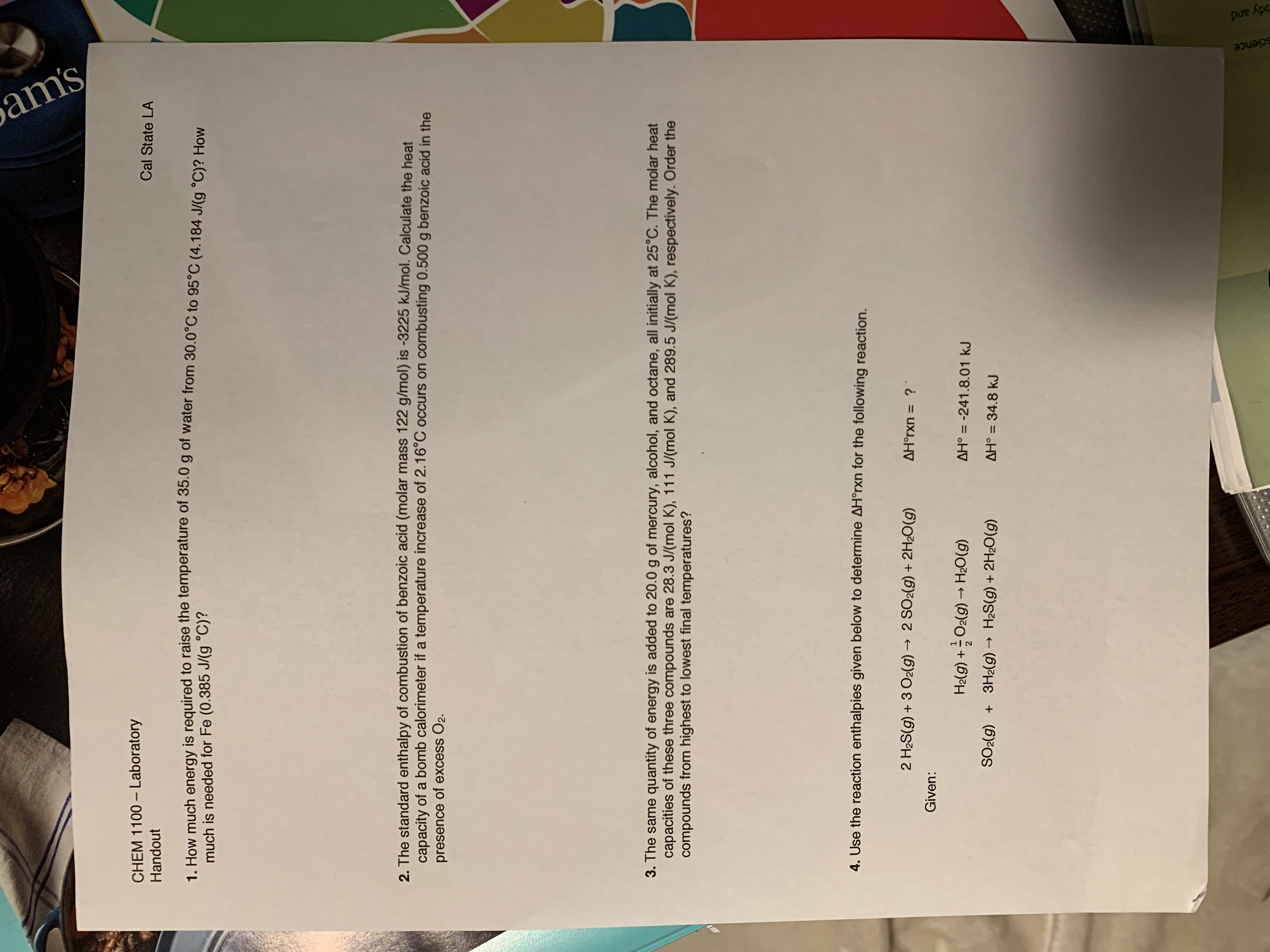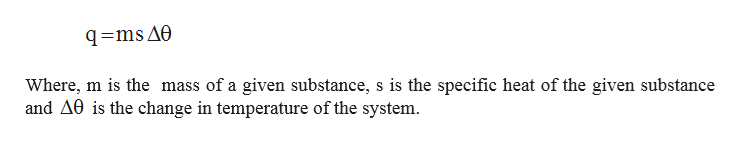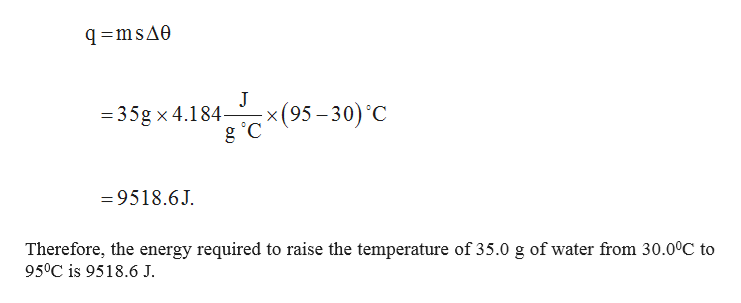# CHEM 1100-LaboratoryCal State LAHandout1. How much energy is required to raise the temperature of 35.0 g of water from 30.0°C to 95°C (4.184 J/(g °C)? Howmuch is needed for Fe (0.385 J/(g °C)?2. The standard enthalpy of combustion of benzoic acid (molar mass 122 g/mol) is -3225 kJ/mol. Calculate the heatcapacity of a bomb calorimeter if a temperature increase of 2.16°C occurs on combusting 0.500 g benzoic acid in thepresence of excess O2.3. The same quantity of energy is added to 20.0g of mercury, alcohol, and octane, all initially at 25°C. The molar heatcapacities of these three compounds are 28.3 J/(mol K), 111 J/ (mol K), and 289.5 J/(mol K), respectively. Order thecompounds from highest to lowest final temperatures?4. Use the reaction enthalpies given below to determine AH°rxn for the following reaction.2 H2S(g)3 O2(g)2 SO2(g) + 2H2O(g)AHorxn = ?Given:H2lg)+O2(g)H2O(g)AH° -241.8.01 kJSO2(g) 3H2(g)H2S(g)2H20(g)AH 34.8 kJ1Scienceody andam's

Question
78 views

I need help on question 1help_outlineImage TranscriptioncloseCHEM 1100-Laboratory Cal State LA Handout 1. How much energy is required to raise the temperature of 35.0 g of water from 30.0°C to 95°C (4.184 J/(g °C)? How much is needed for Fe (0.385 J/(g °C)? 2. The standard enthalpy of combustion of benzoic acid (molar mass 122 g/mol) is -3225 kJ/mol. Calculate the heat capacity of a bomb calorimeter if a temperature increase of 2.16°C occurs on combusting 0.500 g benzoic acid in the presence of excess O2. 3. The same quantity of energy is added to 20.0g of mercury, alcohol, and octane, all initially at 25°C. The molar heat capacities of these three compounds are 28.3 J/(mol K), 111 J/ (mol K), and 289.5 J/(mol K), respectively. Order the compounds from highest to lowest final temperatures? 4. Use the reaction enthalpies given below to determine AH°rxn for the following reaction. 2 H2S(g)3 O2(g)2 SO2(g) + 2H2O(g) AHorxn = ? Given: H2lg)+O2(g)H2O(g) AH° -241.8.01 kJ SO2(g) 3H2(g)H2S(g)2H20(g) AH 34.8 kJ 1 Science ody and am's fullscreen
check_circle

Step 1

The process is to raise the temperature of 35.0 g of water from 30.00C to 950C where the specific heat of liquid water is 4.184 J/ (g 0C). The same process has to be done for Fe also where the specific heat of Fe is 0.385 J/ (g 0C).

The important factor of this process is that only temperature change is involved. Therefore, the appropriate equation to use for the calculation energy required is given below.help_outlineImage TranscriptioncloseWhere, m is the mass of a given substance, s is the specific heat of the given substance and A0 is the change in temperature of the system. fullscreen
Step 2

Calculation for...help_outlineImage Transcriptioncloseq msAe J - x (95-30) C =35g x 4.184- g C =9518.6J Therefore, the energy required to raise the temperature of 35.0 g of water from 30.0°C to 950C is 9518.6 J. fullscreen

### Want to see the full answer?

See Solution

#### Want to see this answer and more?

Solutions are written by subject experts who are available 24/7. Questions are typically answered within 1 hour.*

See Solution
*Response times may vary by subject and question.
Tagged in

### Chemistry